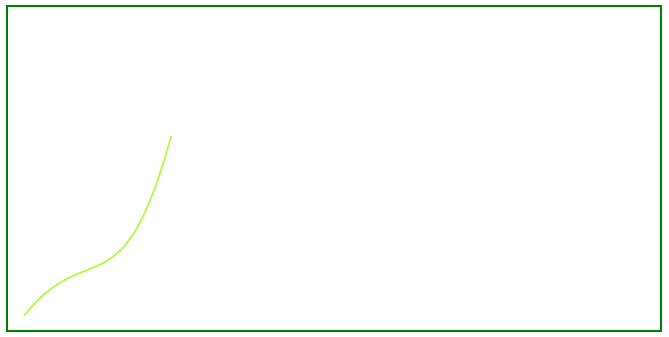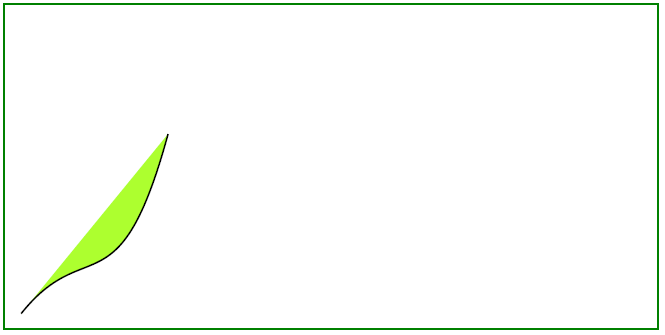# Add Curve Object to PDF file

Contents
[ ]

A graph Curve is a connected union of projective lines, each line meeting three others in ordinary double points.

Aspose.PDF for Java shows how to use Bézier curves in your Graphs. Bézier curves are widely used in computer graphics to model smooth curves. The curve is completely contained in the convex hull of its control points, the points may be graphically displayed and used to manipulate the curve intuitively. The entire curve is contained in the quadrilateral whose corners are the four given points (their convex hull).

In this article, we will investigate simply graph curves, and filled curves, that you can create in your PDF document.

1. Create Document instance.

2. Create Drawing object with certain dimensions.

3. Set Border for Drawing object.

4. Add Graph object to paragraphs collection of page.

``````    public static void ExampleCurve() {
// Create Document instance
Document pdfDocument = new Document();
// Add page to pages collection of PDF file

// Create Drawing object with certain dimensions
Graph graph = new Graph(400, 200);
// Set border for Drawing object
BorderInfo borderInfo = new BorderInfo(BorderSide.All, Color.getGreen());
graph.setBorder(borderInfo);

Curve curve1 = new Curve(new float[] { 10, 10, 50, 60, 70, 10, 100, 120});

curve1.getGraphInfo().setColor(Color.getGreenYellow());

// Add Graph object to paragraphs collection of page

// Save PDF file
}
``````

The following picture shows the result executed with our code snippet:## Create Filled Curve Object

This example shows how to add a Curve object that is filled with color.

``````    public static void ExampleFilledCurve() {
// Create Document instance
Document pdfDocument = new Document();
// Add page to pages collection of PDF file

// Create Drawing object with certain dimensions
Graph graph = new Graph(400, 200);
// Set border for Drawing object
BorderInfo borderInfo = new BorderInfo(BorderSide.All, Color.getGreen());
graph.setBorder(borderInfo);

Curve curve1 = new Curve(new float[] { 10, 10, 50, 60, 70, 10, 100, 120});
curve1.getGraphInfo().setFillColor(Color.getGreenYellow());